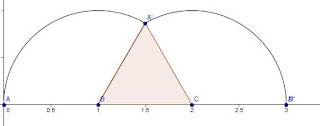## Thursday, 14 March 2013

### Interesting Pi Fact)

The image above shows the locus of points on a rolling circle as it completes one revolution. It was Torricelli who first realized that the area under one arch of this curve was exactly three times the area of the given circle.

Several hundred years later (today) I was reading a post from Math Fun Facts page at Harvey Mudd College.

The very first one on their "most popular" for the day was a neat relation with a similarity to the cycloid fact shown above. It showed that if you roll a square, instead of a circle, and "Each time it comes to a rest, mark the position of the red dot. When the red dot again touches the line, stop. " When you connect these points with a straight lines, they cut off an area with an area exactly three times the area of the original square.
Then they add that, "The same holds for pentagons, hexagons, and any regular n-gon!"
Awesome (but what does that have to do with Pi you ask)..

I suddenly wondered what the area would be if we tracked the actual path of the corner rather than just marking the stopping point.
The firs arc is a circular arc between two adjacent edges so it would be a 90 degree arc with a radius of one side of the square, the distance between the two adjacent points. Easy so far, that part has an area of π /4 times the original square area.
The second arc will have a center on the point opposite the original corner, so it will have a radius equal to the diagonal of the square, and so a 90 degree arc for this will be 2 π/4 times the area of the square.
The final rotation will be another 90 degree rotation with a radius of one side length, so it too will have an area of π/4 times the original square.

And the total of the three areas will be exactly π. If you have read carefully, or if you examine the image of the path below, you will realize that this calculation so far has omitted two small triangles from the total area.These two triangles are each right triangles with legs of one unit, and thus together have the same areas as the original square. So the total area under the arcs is the area of the square added to π times the area of the original square (unless I am overlooking some hideous mathematical mistake, which I sometimes do)...

Maybe the neatest part is that I found this out on Pi day.... Sweet.The image of an equilateral triangle under rotation makes it clear that the area under the arcs is equal to the area of the original plus 2/3 of a circle with the same radius as the original sides of the triangle. Since the area of a unit equilateral triangle is sqrt(3)/4 I can't see much hope for a nice relation with other regular polygons, but will pursue when time allows to see what pops up. If you get time before me (or if this is one of the many things known to almost everyone but me) please advise.

I'm adding the rotation of the regular Pentagon here.In it, as in the triangle and square, it assumes that we get (n-1) arcs of degree 360/n with radii equal to the lengths of the chords from all the other n-1 points back to the point p we trace for our locus.  It is also true that the original figure seems to be equal to the missing area due to moving the center of radius from point to point.
My calculation of the area of the unit Pentagon was π times[3 2/5 + sqrt(5)].
For the moment it seems that the area is getting farther and farther from 3.  I Think I'm moving over to playing with polygons with a unit area which may make the calculations a little crazy. Out on a wing here looking for something pretty.